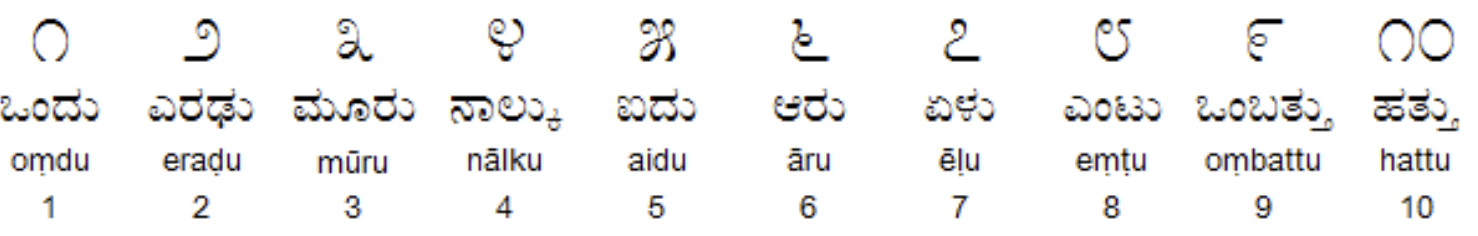Want to share your content on R-bloggers? click here if you have a blog, or here if you don't.

Kannada MNIST dataset is another MNIST-type Digits dataset for Kannada (Indian) Language. All details of the dataset curation has been captured in the paper titled: “Kannada-MNIST: A new handwritten digits dataset for the Kannada language.” by Vinay Uday Prabhu. The github repo of the author can be found here.The objective of this post is to demonstrate how to use `h2o.ai`’s `automl` function to quickly get a (better) baseline. Thsi also proves a point how these `automl` tools help democratizing Machine Learning Model Building process.

• `h2o` – for Machine Learning
• `tidyverse` – for Data Manipulation
```library(h2o)
library(tidyverse)```

### Initializing H2O Cluster

`h2o::h2o.init()`

```train <- read_csv("~/Documents/R Codes/Kannada-MNIST/train.csv")

### Checking the shape / dimension of the dataframe

`dim(train)`

784 Pixel Values + 1 Label denoting what digit it’s.

### Label Count

`train  %>% count(label)`

### Visualizing the Kannada MNIST Digits

```# visualize the digits
par(mfcol=c(6,6))

par(mar=c(0, 0, 3, 0), xaxs='i', yaxs='i')

for (idx in 1:36) {

im<-matrix((train[idx,2:ncol(train)]), nrow=28, ncol=28)

im_numbers <- apply(im, 2, as.numeric)

image(1:28, 1:28, im_numbers, col=gray((0:255)/255), main=paste(train\$label[idx]))
}```### Converting R dataframe to H2O object which is required by H2O functions

```train_h <- as.h2o(train)
test_h <- as.h2o(test)
valid_h <- as.h2o(valid)```

### Converting our numeric target variable into a factor for the algorithm to perform Classification

```train_h\$label <- as.factor(train_h\$label)
valid_h\$label <- as.factor(valid_h\$label)```

### Explanatory and Response Variables

```x <- names(train)[-1]
y <- 'label'```

### AutoML in Action

```aml <- h2o::h2o.automl(x = x,
y = y,
training_frame = train_h,
nfolds = 3,
max_runtime_secs = 1000)```

`nfolds` denotes the number of folds for cross-validation and `max_runtime_secs` represents the maximum amount of time the AutoML process can go on.

Leaderboard is where the AutoML lists the top performing Models.

`[email protected]`

### Prediction and Submission

```pred <- h2o.predict(aml, test_h)

submission\$label <- as.vector(pred\$predict)

#write_csv(submission, "submission_automl.csv")```

### Submission (for Kaggle)

`write_csv(submission, "submission_automl.csv")`

This is currently a playground Competition on Kaggle. So, this submission file can be submitted to this competition. Based on the above parameters the submission scored `0.90720` in the public leaderboard. `0.90` score in an MNIST Classification is close to nothing, but I hope this code snippet can serve as quick starter template for anyone attempting to begin with AutoML.